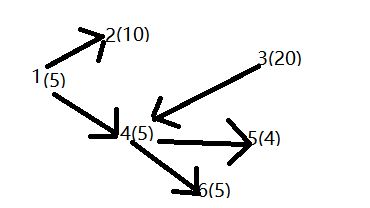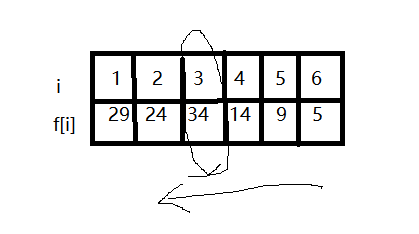# 第三章算法作业

## 1. 任选作业题”单调递增最长子序列“、”挖地雷“、”编辑距离问题“中的一题分析。

3-3 挖地雷 (25分)

### 输入格式:

xi yi //表示从xi可到yi，xi<yi。

### 输出格式:

k1-k2−…−kv //挖地雷的顺序 挖到最多的雷。

### 输入样例:

6
5 10 20 5 4 5
1 2
1 4
2 4
3 4
4 5
4 6
5 6
0 0


### 输出样例:

3-4-5-6
34
#include<iostream>
#include<cstring>
#define MAXN 200
using namespace std;
bool a[MAXN][MAXN];//a[i][j]表示第i个地窖和第j个地窖是否通路
int w[MAXN];//每个地窖的地雷数
int f[MAXN];//f[i]表示从第i个地窖开始挖的最多地雷数
int suf[MAXN];
int main()
{
long n, i, j, x, y, l, k, maxn;
cin >> n;
for(i = 1; i <= n; i++)
{
cin >> w[i];
}
while(cin >> x >> y)
{
if(x == 0 && y == 0)
break;
a[x][y] = true;
}
f[n] = w[n];//初始状态
for(i = n - 1; i >= 1; i--)
{
l = 0;
k = 0;
for(j = i + 1; j <= n; j++)
{
if(a[i][j] && f[j] > l)
{
l = f[j];
k = j;
}
}
f[i] = w[i] + l;
suf[i] = k;
}
k = 1;
for(i = 1;i <= n; i++)//从n个数中找最大值
{
if(f[i] > f[k])
k = i;
}
maxn = f[k];
cout << k;//先输出起始点
k = suf[k];
while(k != 0)
{
cout << "-" <<k;
k = suf[k];
}
cout << endl;
cout << maxn << endl;
return 0;
} 

 

#### 1.1 根据最优子结构性质，列出递归方程式

f[i] = max{w[i] + f[j]} (a[i][j] > 0, i< j <= n)

#### 1.2 给出填表法中表的维度、填表范围和填表顺序## 3. 说明结对编程情况

posted @ 2020-10-27 21:31  林冬璇  阅读(181)  评论(0编辑  收藏  举报